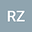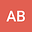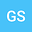One-Dimensional Flow to a Constant-Pressure Boundary in a Pressure-Sensitive Porous Medium
•••Amarjot Bhullar
Imperial College London
Author ProfileGospel Stewart
Imperial College London
Author Profile## Abstract

In some “pressure-sensitive” rock formations, the variation of permeability with pore pressure is sufficiently large that it needs to be accounted for. Accounting for this variation of permeability renders the pressure diffusion equation nonlinear, and not amenable to exact analytical solutions. A constitutive model that fits many data sets is that of a permeability that varies exponentially with pressure. One-dimensional flow to a constant-pressure boundary in a medium whose permeability varies exponentially with pressure is investigated in this work, using numerical and approximate approaches. As part of the analysis, a dimensionless “pressure-sensitivity” parameter β is defined as the logarithm of the ratio of the far-field permeability to the permeability at the outflow boundary. The problem can be approached by applying a Boltzmann transformation to the governing PDE, and converting the resultant ODE into an integral equation, which can be solved by an iterative procedure. This numerical solution can serve as a benchmark against which to judge the accuracy of approximate solutions. One simple approximate model is based on the “sorptivity approximation” developed by Philip for unsaturated flow, which can be adapted to any nonlinear diffusion problem. This model linearizes the problem by defining an effective permeability as a drawdown-weighted average of the permeability as a function of pore pressure. The Philip approximation predicts that the flowrate, normalized against the case in which the permeability is constant, is 1–0.333β, to first-order in β. This problem can also be approached as perturbation problem, with β as the perturbation parameter. The zeroth-order solution is the classical error-function solution for a 1D diffusion problem with a constant-pressure boundary. The first-order correction is obtained in closed form, from which the flowrate at the outflow boundary is found. To first-order, the normalized flowrate is 1-0.318β. This solution is extended to higher values of β by defining an effective permeability such that, if inserted into the analytical solution for the mathematically linear problem, yields a flowrate that is exact to first order in β. When compared to the numerical solution, this new result has 5% accuracy out to values of β = 2, which probably covers most cases of interest.